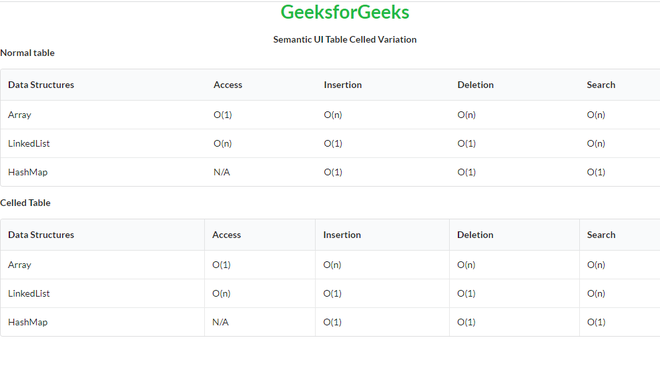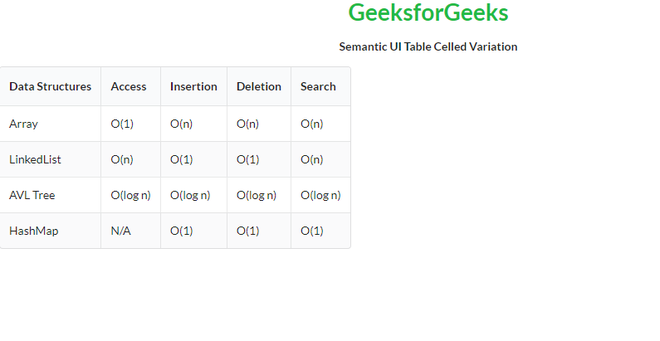# Semantic-UI Table Celled Variation

Semantic UI is an open-source framework that uses CSS and jQuery to build great user interfaces. It is the same as a bootstrap for use and has great different elements to use to make your website look more amazing. It uses a class to add CSS to the elements. Tables are an easy way to organize a lot of data. A table is an arrangement of data in rows and columns, or possibly in a more complex structure. Tables are widely used in communication, research, and data analysis. Tables are useful for various tasks such as presenting text information and numerical data. It can be used to compare two or more items in the tabular form layout. Tables are used to create databases. An HTML table and a Semantic UI table both are the same structurally.

In the case of Table Celled Variation, a table has each row divided into separate cells.

Semantic UI Table Celled Variation Classes:

• table: This class creates a table in Semantic UI.
• celled: This class divides each row of our table into separate cells.

Syntax:

```<table class="ui celled table">
<tr>
<td></td>
...
</tr>
...
</table>```

Example 1: This is a basic example illustrating Table Celled Variation  made using Semantic UI.

## HTML

 ` ` `<``html``> ` ` `  `<``head``> ` `    ``<``title``>Semantic UI Table Celled Variation ` `    ``<``link` `href``= ` `    ``"https://cdnjs.cloudflare.com/ajax/libs/semantic-ui/2.4.1/semantic.min.css"`  `    ``rel``=``"stylesheet"` `/> ` `    ``<``script` `src``=``"https://code.jquery.com/jquery-3.1.1.min.js"` `        ``integrity``=``"sha256-hVVnYaiADRTO2PzUGmuLJr8BLUSjGIZsDYGmIJLv2b8="` `         ``crossorigin``=``"anonymous"``>  ` `    `` ` `    ``<``script` `src``= ` `"https://cdnjs.cloudflare.com/ajax/libs/semantic-ui/2.4.1/semantic.min.js"``> ` `    `` ` ` ` ` `  `<``body``> ` `    ``<``center``> ` `        ``<``h1` `class``=``"ui green header"``>GeeksforGeeks ` `        ``<``strong``>Semantic UI Table Celled Variation ` `    `` ` `    ``<``b``>Normal table ` `    ``<``table` `class``=``"ui table"``> ` `        ``<``thead``> ` `            ``<``tr``> ` `                ``<``th``>Data Structures ` `                ``<``th``>Access ` `                ``<``th``>Insertion ` `                ``<``th``>Deletion ` `                ``<``th``>Search ` `            `` ` `        `` ` `        ``<``tbody``> ` `            ``<``tr``> ` `                ``<``td``>Array ` `                ``<``td``>O(1) ` `                ``<``td``>O(n) ` `                ``<``td``>O(n) ` `                ``<``td``>O(n) ` `            `` ` `            ``<``tr``> ` `                ``<``td``>LinkedList ` `                ``<``td``>O(n) ` `                ``<``td``>O(1) ` `                ``<``td``>O(1) ` `                ``<``td``>O(n) ` `            `` ` `            ``<``tr``> ` `                ``<``td``>HashMap ` `                ``<``td``>N/A ` `                ``<``td``>O(1) ` `                ``<``td``>O(1) ` `                ``<``td``>O(1) ` `            `` ` `        `` ` `    `` ` `    ``<``b``>Celled Table ` `    ``<``table` `class``=``"ui celled table"``> ` `        ``<``thead``> ` `            ``<``tr``> ` `                ``<``th``>Data Structures ` `                ``<``th``>Access ` `                ``<``th``>Insertion ` `                ``<``th``>Deletion ` `                ``<``th``>Search ` `            `` ` `        `` ` `        ``<``tbody``> ` `            ``<``tr``> ` `                ``<``td``>Array ` `                ``<``td``>O(1) ` `                ``<``td``>O(n) ` `                ``<``td``>O(n) ` `                ``<``td``>O(n) ` `            `` ` `            ``<``tr``> ` `                ``<``td``>LinkedList ` `                ``<``td``>O(n) ` `                ``<``td``>O(1) ` `                ``<``td``>O(1) ` `                ``<``td``>O(n) ` `            `` ` `            ``<``tr``> ` `                ``<``td``>HashMap ` `                ``<``td``>N/A ` `                ``<``td``>O(1) ` `                ``<``td``>O(1) ` `                ``<``td``>O(1) ` `            `` ` `        `` ` `    `` ` ` ` ` `  ``

Output:Example 2: This is a basic example illustrating Collapsed Striped Table Celled Variation  made using Semantic UI.

## HTML

 ` ` `<``html``> ` ` `  `<``head``> ` `    ``<``title``>Semantic UI Table Celled Variation ` `    ``<``link` `href``= ` `"https://cdnjs.cloudflare.com/ajax/libs/semantic-ui/2.4.1/semantic.min.css"`  `    ``rel``=``"stylesheet"` `/> ` `    ``<``script` `src``= ` `    ``"https://code.jquery.com/jquery-3.1.1.min.js"` `        ``integrity``= ` `        ``"sha256-hVVnYaiADRTO2PzUGmuLJr8BLUSjGIZsDYGmIJLv2b8="`  `        ``crossorigin``=``"anonymous"``> ` `    ``<``script` `src``= ` `"https://cdnjs.cloudflare.com/ajax/libs/semantic-ui/2.4.1/semantic.min.js"``> ` `    `` ` ` ` ` `  `<``body``> ` `    ``<``center``> ` `        ``<``h1` `class``=``"ui green header"``>GeeksforGeeks ` `        ``<``strong``>Semantic UI Table Celled Variation ` `    `` ` `    ``<``table` `class``=``"ui celled collapsing striped table"``> ` `        ``<``thead``> ` `            ``<``tr``> ` `                ``<``th``>Data Structures ` `                ``<``th``>Access ` `                ``<``th``>Insertion ` `                ``<``th``>Deletion ` `                ``<``th``>Search ` `            `` ` `        `` ` `        ``<``tbody``> ` `            ``<``tr``> ` `                ``<``td``>Array ` `                ``<``td``>O(1) ` `                ``<``td``>O(n) ` `                ``<``td``>O(n) ` `                ``<``td``>O(n) ` `            `` ` `            ``<``tr``> ` `                ``<``td``>LinkedList ` `                ``<``td``>O(n) ` `                ``<``td``>O(1) ` `                ``<``td``>O(1) ` `                ``<``td``>O(n) ` `            `` ` `            ``<``tr``> ` `                ``<``td``>AVL Tree ` `                ``<``td``>O(log n) ` `                ``<``td``>O(log n) ` `                ``<``td``>O(log n) ` `                ``<``td``>O(log n) ` `            `` ` `            ``<``tr``> ` `                ``<``td``>HashMap ` `                ``<``td``>N/A ` `                ``<``td``>O(1) ` `                ``<``td``>O(1) ` `                ``<``td``>O(1) ` `            `` ` `        `` ` `    `` ` ` ` ` `  ``

Output:Whether you're preparing for your first job interview or aiming to upskill in this ever-evolving tech landscape, GeeksforGeeks Courses are your key to success. We provide top-quality content at affordable prices, all geared towards accelerating your growth in a time-bound manner. Join the millions we've already empowered, and we're here to do the same for you. Don't miss out - check it out now!